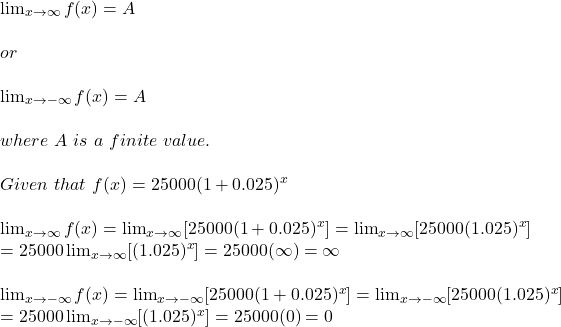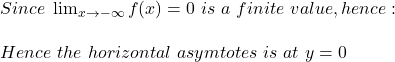What is the equation of the line that represents the horizontal asymptote of the function f(x)=25,000(1+0.025)^(x)?

Question

What is the equation of the line that represents the horizontal asymptote of the function f(x)=25,000(1+0.025)^(x)?

in progress 0
5 months 2021-08-18T06:22:03+00:00 1 Answers 7 views 0